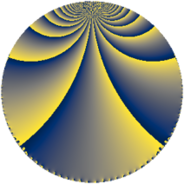# Properties

 Label 252.4.bfLevel $252$ Weight $4$ Character orbit 252.bf Rep. character $\chi_{252}(19,\cdot)$ Character field $\Q(\zeta_{6})$ Dimension $116$ Sturm bound $192$

# Learn more

## Defining parameters

 Level: $$N$$ $$=$$ $$252 = 2^{2} \cdot 3^{2} \cdot 7$$ Weight: $$k$$ $$=$$ $$4$$ Character orbit: $$[\chi]$$ $$=$$ 252.bf (of order $$6$$ and degree $$2$$) Character conductor: $$\operatorname{cond}(\chi)$$ $$=$$ $$28$$ Character field: $$\Q(\zeta_{6})$$ Sturm bound: $$192$$

## Dimensions

The following table gives the dimensions of various subspaces of $$M_{4}(252, [\chi])$$.

Total New Old
Modular forms 304 124 180
Cusp forms 272 116 156
Eisenstein series 32 8 24

## Trace form

 $$116q + 2q^{2} + 4q^{4} + 6q^{5} + 80q^{8} + O(q^{10})$$ $$116q + 2q^{2} + 4q^{4} + 6q^{5} + 80q^{8} - 12q^{10} - 86q^{14} - 44q^{16} + 6q^{17} + 344q^{22} + 1332q^{25} + 354q^{26} - 452q^{28} - 448q^{29} - 68q^{32} - 246q^{37} + 126q^{38} + 792q^{40} - 220q^{44} + 352q^{46} + 844q^{49} - 1628q^{50} + 108q^{52} + 574q^{53} + 1352q^{56} - 576q^{58} + 294q^{61} - 1112q^{64} - 156q^{65} - 1368q^{68} + 740q^{70} - 978q^{73} - 822q^{74} + 2314q^{77} - 1056q^{80} - 3360q^{82} - 388q^{85} + 130q^{86} - 1312q^{88} + 3186q^{89} - 2360q^{92} + 2184q^{94} - 2996q^{98} + O(q^{100})$$

## Decomposition of $$S_{4}^{\mathrm{new}}(252, [\chi])$$ into newform subspaces

The newforms in this space have not yet been added to the LMFDB.

## Decomposition of $$S_{4}^{\mathrm{old}}(252, [\chi])$$ into lower level spaces

$$S_{4}^{\mathrm{old}}(252, [\chi]) \cong$$ $$S_{4}^{\mathrm{new}}(28, [\chi])$$$$^{\oplus 3}$$$$\oplus$$$$S_{4}^{\mathrm{new}}(84, [\chi])$$$$^{\oplus 2}$$